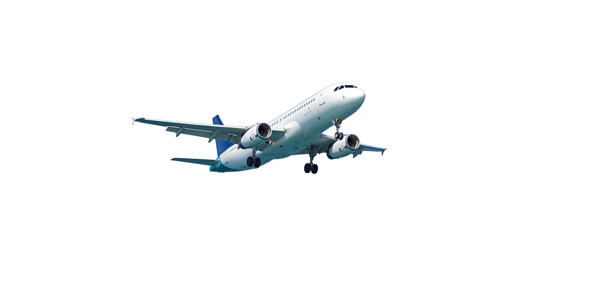# How Much Do You Know About Physics And Paper Airplanes (Advanced)

Approved & Edited by ProProfs Editorial Team
The editorial team at ProProfs Quizzes consists of a select group of subject experts, trivia writers, and quiz masters who have authored over 10,000 quizzes taken by more than 100 million users. This team includes our in-house seasoned quiz moderators and subject matter experts. Our editorial experts, spread across the world, are rigorously trained using our comprehensive guidelines to ensure that you receive the highest quality quizzes.
| By Foldingtofly
F
Foldingtofly
Community Contributor
Quizzes Created: 1 | Total Attempts: 136
Questions: 10 | Attempts: 136Settings• 1.

### What is the function of a fuselage in an airplane?

• A.

Generates Thrust

• B.

Changes Lift and Drag

• C.

Control Direction of Plane

• D.

Controls Pitch

• E.

None of the above

E. None of the above
Explanation
The function of a fuselage in an airplane is not mentioned in the given options. The fuselage is the main body structure of an aircraft that accommodates the crew, passengers, and cargo. It also houses the wings, tail, and engines. The fuselage provides structural integrity to the aircraft and houses various systems and components necessary for flight, such as fuel tanks, landing gear, and control surfaces. Therefore, none of the given options accurately describe the function of a fuselage.

Rate this question:

• 2.

### Which part of the plane is considered the most important and why so?

• A.

Wings, because they are the main generator of the plane's lift.

• B.

Fuselage, because they help to generate kinetic engine for the plane to function

• C.

Tail, because they help to control the airplane's thrust.

• D.

Elevator, because they lessen air resistance for the plane to fly smoothly.

A. Wings, because they are the main generator of the plane's lift.
Explanation
The wings of a plane are considered the most important because they are responsible for generating the lift that allows the plane to stay in the air. Lift is created by the shape of the wings and the flow of air over them. Without the wings, the plane would not be able to generate enough lift to overcome gravity and stay airborne. The other options mentioned, such as the fuselage, tail, and elevator, all play important roles in the plane's overall functionality, but they do not have the same direct impact on generating lift as the wings do.

Rate this question:

• 3.

### What are the two primary forces that pulls the plane down?(Hint:

• A.

Weight, Gravity

• B.

Lift, Thrust

• C.

Potential Energy, Weight

• D.

Gravity, Lift

• E.

None of the above

A. Weight, Gravity
Explanation
Weight and gravity are the two primary forces that pull the plane down. Weight is the force exerted by the mass of the plane due to gravity. Gravity is the natural force that pulls objects towards the center of the Earth. These two forces act in the downward direction, causing the plane to be pulled down towards the ground. Lift and thrust are not forces that pull the plane down, so they are not the correct answer.

Rate this question:

• 4.

### What is air resistance?

• A.

Drag caused by water particles surrounding your object

• B.

Friction caused by surface of object and air particles surrounding it.

• C.

Air particles that collides against object surface causing resistance to drag.

• D.

The opposite motion of thrust.

B. Friction caused by surface of object and air particles surrounding it.
Explanation
Air resistance refers to the frictional force that acts on an object as it moves through the air. It is caused by the interaction between the surface of the object and the air particles surrounding it. As the object moves, the air particles collide with its surface, creating resistance or drag. This drag force opposes the motion of the object and can affect its speed and trajectory. Therefore, the correct answer is "Friction caused by surface of object and air particles surrounding it."

Rate this question:

• 5.

### What is the equation for calculating force?

• A.

Force=Weight X Gravity

• B.

Force= Thrust/Weight

• C.

Force=Mass X Acceleration

• D.

Force= (Acceleration/Drag) x Distance

C. Force=Mass X Acceleration
Explanation
The equation for calculating force is Force=Mass X Acceleration. This equation is derived from Newton's second law of motion, which states that the force acting on an object is equal to the mass of the object multiplied by its acceleration. This equation is widely used in physics to determine the force exerted on an object in various situations.

Rate this question:

• 6.

• 7.

• 8.

• 9.

• 10.

### Watch the video below. Observe the phenomenon at work here and explain why it is possible for it to occur.

Related TopicsBack to top